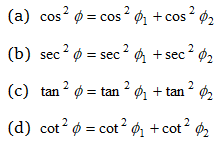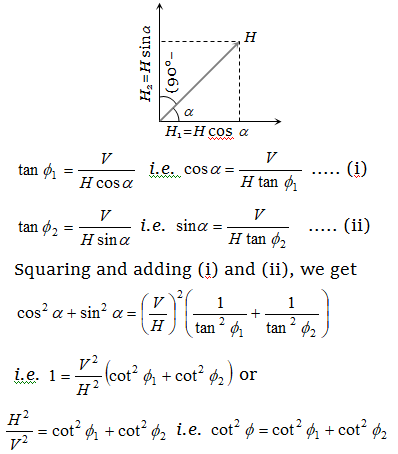# NEET Questions Solved

If ${\varphi }_{1}$ and ${\varphi }_{2}$ be the angles of dip observed in two vertical planes at right angles to each other and $\varphi$ be the true angle of dip, thenLet $\alpha$ be the angle which one of the planes make with the magnetic meridian the other plane makes an angle $\left(90°-\alpha \right)$ with it. The components of H in these planes will be H cos α and H sin α respectively. If ${\varphi }_{1}$ and ${\varphi }_{2}$ are the apparent dips in these two planes, thenThis is the required result.

Difficulty Level:

• 9%
• 2%
• 12%
• 80%